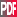12th Conference on Cloud Physics

14.4

Stereophotography of rain drops and compound poisson - cascade processes

S. Lovejoy, McGill University, Montreal, QC, Canada; and D. Schertzer

Precipitation and clouds are fundamentally point-like but when averaged over larger enough scales are usually considered to be continuous and are mathematically modeled using fields (or densities of measures). Until recently when it has been essential to have a particle description, the latter have modeled using uniform Poisson processes in which the number density of particles is considered uniform in space and/or time. Since empirical drop distributions (typpically measured with disdrometers) are never spatially or temporally homogeneous, numerous attempts have been made to model the heterogeneity using classical compound Poisson processes in which heterogeneity over narrow ranges of scales “control” the Poisson process.

While the disdrometer based experiments have increasingly recognized the importance of drop heterogeneity, there has been a growing consensus – at least over the smaller scales – that atmospheric turbulence can be accurately modeled by cascade processes in which energy and other conservative fluxes are concentrated into a hierarchy sparse fractal sets, (more precisely, multifractal measures) in which the heterogeneity occurs over huge ranges of space-time scales in a power law manner. Since the turbulent wind and the drops are strongly coupled, it is natural to suppose that the drop heterogeneity also occurs over wide ranges, and that the latter is largely “controlled” by the turbulence . Recently [Lovejoy, et al., 2003], [Lilley, et al., 2005] the connection between the drop statistics and (Corrsin-Obukov) turbulence has been directly made thanks to the use of stereophotography of rain drops in 10m**3 volumes (the “HYDROP” experiment [Desaulniers-Soucy., et al., 2001], see e.g. fig. 1).

In this talk, we show how the turbulence and particle processes can be combined in a nonclassical compound Poisson-cascade process and we verify the result on the HYDROP data. The key is the liquid water density ρ variance flux (χ) which - following the HYDROP observations and Corrsin-Obukhov passive scalar theory – is conserved from scale to scale (it is the basic multifractal field). The link to the particle description is via the particle number density (n); we show how this can be determined from ? and the turbulent energy flux ε we theoretically predict a k**-5/3 spectrum for ρ and a k**-2 spectrum for n which we confirm is close to observations.

In order to perform simulations respecting these turbulence constraints we start with cascade models of χ and ε cut-off by viscosity at the dissipation scale (roughly 1cm). From these fluxes we determine ρ and n by fractional integration. At scales below 10cm or so, there is typically only one drop in the corresponding sphere; we interpret n as the number density of a (compound) Poisson process and randomly determine the positions of the ith particle: ri. The masses mi, are determined from a unit exponential (Marshall-Palmer) random variable Ei: mi=Ei ρ(ri)/n(ri). The resulting measure (mi, ri) has the observed energy spectrum, the observed multifractal statistics (including the transition from particle scales to field scales) it also has realistic probability (fat tailed, power law) distributions for total mass in a large sphere M. In this case, it predicts a power law with exponent qD=3 (this is an exact result coming from theory and dimensional analysis). We show both on numerical simulations and on the HYDROP data that this prediction is accurately obeyed. In addition, it potentially explains numerous reports that qD=3 for the rain rate. Since it incorporates (in a highly inhomogeneous framework) the Marshall-Palmer exponential drop distribution as well as a Poisson particle process, it bridges the gap between classical and turbulence approaches.

Numerical simulations spanning the range 1cm to 1000km can be readily produced. These simulations can be used for simulating radar reflectivity factors, effective radar reflectivity factors; extensions of the model can be used to simulate rain rates and rain gauges. These models can thus potentially solve various precipitation observer problems.

Fig. 1: An intercomparison of the Compound Poisson-Cascade model (left) with Hydrop drop data (right) only the largest 2,000 (10%) of the drops are shown form clarity. The scale is in meters.

References: Desaulniers-Soucy., N., et al. (2001), The continuum limit in rain and the HYDROP experiment, Atmos. Resear., 59-60, 163-197. Lilley, M., et al. (2005), Multifractal large number of drops limit in Rain, J. Hydrol., (in press). Lovejoy, S., et al. (2003), Large particle number limit in Rain, Phys. Rev. E, 68, 025301.Extended Abstract (1.9M)

Session 14, Precipitation II
Friday, 14 July 2006, 10:30 AM-12:15 PM, Ballroom AD

Previous paper  Next paper

Browse or search entire meeting